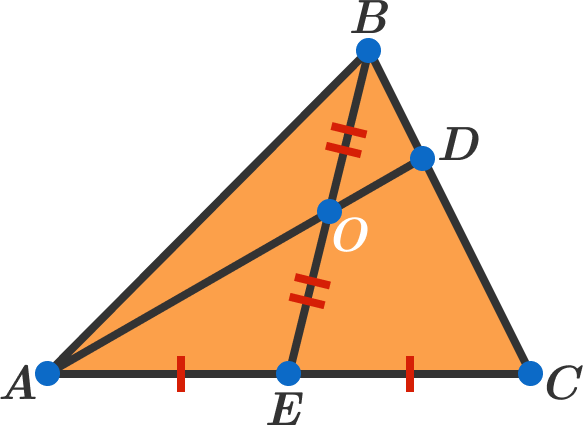# Think about two folds

Geometry Level 3

In $\triangle ABC$, $BE$ is a median and $O$ is the midpoint of $BE$. The line joining $A$ and $O$ meets $BC$ at $D$. Find the ratio of the lengths $AO : OD$.×

Problem Loading...

Note Loading...

Set Loading...